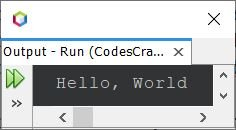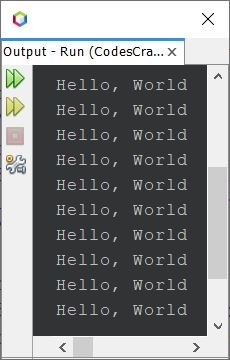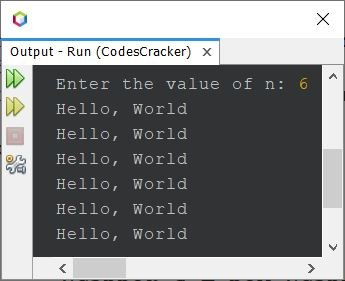# Java Program to Print Hello World

This article covers a program in Java that prints Hello, World. To print Hello, World on the output screen in Java programming, just place the string "Hello, World" inside System.out.println() as shown in the program given below.

## Print Hello World in Java

The question is, write a Java program to print Hello, World. The program given below is its answer:

```public class CodesCracker
{
public static void main(String[] args)
{
System.out.println("Hello, World");
}
}```

When the above Java Program is compiled and executed, it will produce the following output:## Print Hello World in Java 10 Times using for Loop

This program prints Hello, World 10 number of times, using for loop.

```public class CodesCracker
{
public static void main(String[] args)
{
for(int i=0; i<10; i++)
System.out.println("Hello, World");
}
}```

The snapshot given below shows the sample output produced by above Java program on printing of Hello World 10 times:## Print Hello World in Java 10 Times using while Loop

This is the same program as of previous, but created using while loop, instead of for.

```public class CodesCracker
{
public static void main(String[] args)
{
int i=0;
while(i<10)
{
System.out.println("Hello, World");
i++;
}
}
}```

This program produces exactly same output as of previous program. Above program can also be created in this way:

```public class CodesCracker
{
public static void main(String[] args)
{
int i=0;
while(i++<10)
System.out.println("Hello, World");
}
}```

That is, in the code:

`i++<10`

the current value of i gets used, then incremented, as in i++, the ++ is the post increment operator.

## Print Hello World n Times

Now this program prints n number of times, the string Hello, World. For example, if the value of n is 100, then this program prints 100 Hello, World. The value of n is received by user at run-time of the program, using Scanner class.

```import java.util.Scanner;

public class CodesCracker
{
public static void main(String[] args)
{
int n;
Scanner s = new Scanner(System.in);

System.out.print("Enter the value of n: ");
n = s.nextInt();

for(int i=0; i<n; i++)
System.out.println("Hello, World");
}
}```

The snapshot given below shows the sample run of above program with user input 6 as value of n to print 6 Hello, World## Print Hello World in Java using Function

To print Hello, World in Java using user-defined function, use following program:

```public class CodesCracker
{
public static void main(String[] args)
{
HelloWorld();
}
public static void HelloWorld()
{
System.out.println("Hello, World");
}
}```

The output of this program is:

`Hello, World`

## Print Hello World in Java using Class

To print Hello, World in Java using class and object, use following program:

```public class CodesCracker
{
public static void main(String[] args)
{
CodesCracker cc = new CodesCracker();
cc.HelloWorld();
}
public static void HelloWorld()
{
System.out.println("Hello, World");
}
}```

This program produces exactly same output as of previous program.

## Print Hello World in Java using Constructor

This is the last program of this article, created using a constructor. Constructor gets automatically called after the creation of an object of the class, in which the constructor is defined.

```public class CodesCracker
{
CodesCracker()
{
System.out.println("Hello, World");
}
public static void main(String[] args)
{
CodesCracker cc = new CodesCracker();
}
}```

#### Same Program in Other Languages

Java Online Test

« Previous Program Next Program »